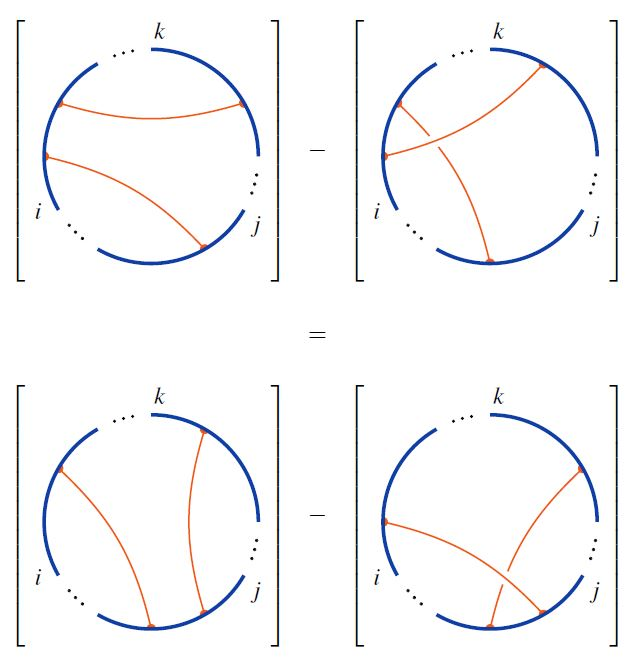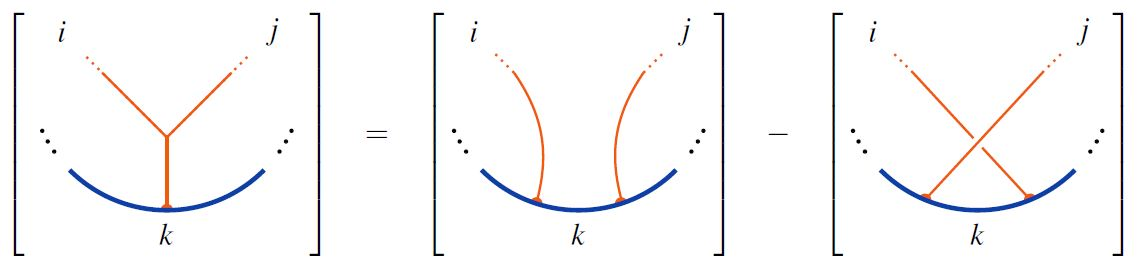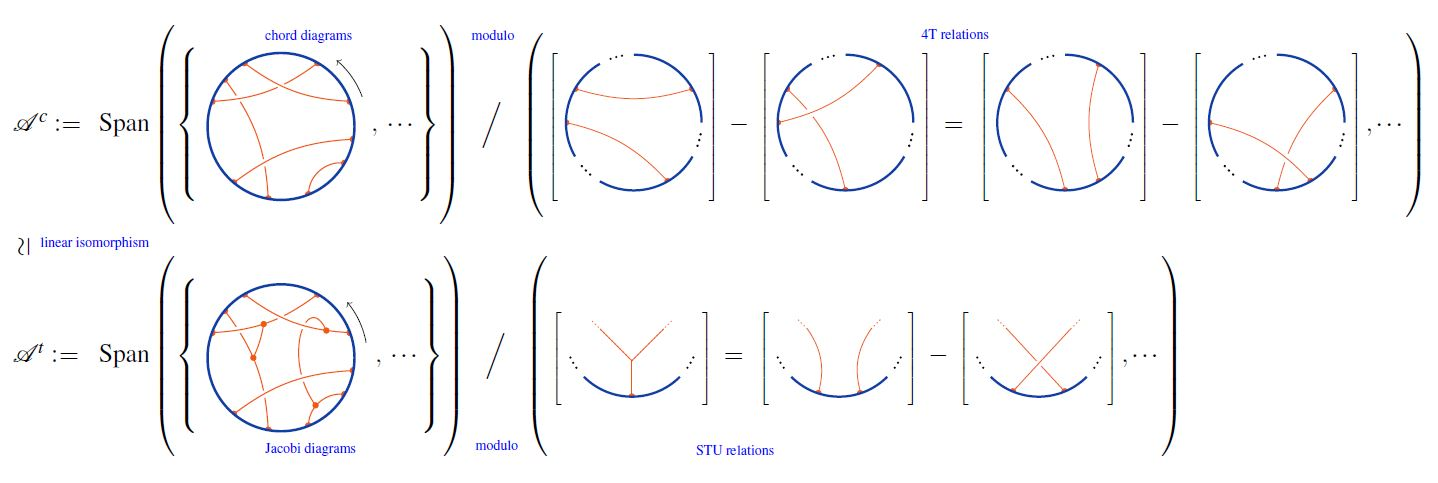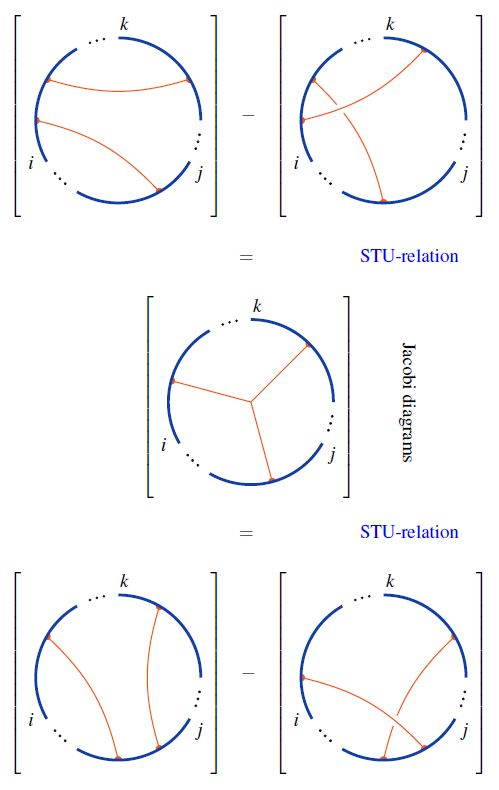# nLab chord diagrams modulo 4T are Jacobi diagrams modulo STU

Contents

### Context

#### Knot theory

knot theory

Examples/classes:

Types

knot invariants

Related concepts:

category: knot theory

# Contents

## Ingredients

Consider

1. the set $\mathcal{D}^c$ of chord diagrams;

2. the set $\mathcal{D}^t$ of Jacobi diagrams;

Write

(1)$\mathcal{D}^c \overset{i}{\hookrightarrow} \mathcal{D}^t$

for the canonical injection, regarding chord diagrams as Jacobi diagrams without internal vertices, hence with all vertices on the circle.

For $R \in$ CRing a commutative ring, write

1. $R\langle \mathcal{D}^c \rangle$ for the $R$-linear span of chord diagrams;

2. $R\langle \mathcal{D}^t \rangle$ for the $R$linear span of Jacobi diagrams

and finally

(2)$\mathcal{A}^c \;\coloneqq\; R\langle \mathcal{D}^c \rangle/4T \,, \phantom{AAAA} \mathcal{A}^t \;\coloneqq\; R\langle \mathcal{D}^t\rangle/STU$

for the respective quotient spaces by the 4T relationsand by the STU relationsrespectively.

graphics from Sati-Schreiber 19c

## Statement

The linear extension of the canonical inclusion $\mathbb{D}^c \overset{i}{\hookrightarrow} \mathbb{D}^t$ (1) descends to the quotients (2) and yields a linear isomorphism:

$\mathcal{A}^c \underoverset{\simeq}{i}{\longrightarrow} \mathcal{A}^t$This is due to Bar-Natan 95, Theorem 6. See also Chmutov-Duzhin-Mostovoy 11, 5.3

graphics from Sati-Schreiber 19

The key step of the proof is to observe that the STU-relations imply the 4T-relations as follows:graphics from Sati-Schreiber 19c

## Application

### Relation to weight systems

The immediate consequence is that the space of framed weight systems $\mathcal{W}$, which by definition is the dual vector space to the linear span of chord diagrams modulo the 4T-relations, is equiuvalently also the dual vector space to the linear span of chord diagrams modulo the 4T-relations:

\begin{aligned} \mathcal{W} & \coloneqq (\mathcal{A}^c)^\ast \\ & \simeq (\mathcal{A}^t)^\ast \end{aligned}

The result is due to

Lecture notes

Textbook accounts: